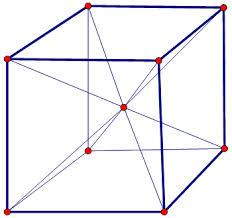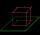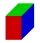# Center of the cube

The Center of the cube has a distance 16 cm from each vertex.

Calculate the volume V and surface area S of the cube.

V =  6306.2 cm3
S =  2048 cm2

### Step-by-step explanation:Did you find an error or inaccuracy? Feel free to write us. Thank you!Tips to related online calculators
Tip: Our volume units converter will help you with the conversion of volume units.
Pythagorean theorem is the base for the right triangle calculator.

#### You need to know the following knowledge to solve this word math problem:

We encourage you to watch this tutorial video on this math problem:

## Related math problems and questions:

• Cube diagonalsDetermine the volume and surface area of the cube if you know the length of the body diagonal u = 216 cm.
• Body diagonal - cubeCalculate the surface and cube volume with body diagonal 15 cm long.
• Space diagonalThe space diagonal of a cube is 129.91 mm. Find the lateral area, surface area and the volume of the cube.
• Body diagonalFind the cube surface if its body diagonal has a size of 6 cm.
• Surface and volume - cubeFind the surface and volume of a cube whose wall diagonal is 5 cm long.
• Cube wallCalculate the cube's diagonal if you know that one wall's surface is equal to 36 centimeters square. Please also calculate its volume.
• Cube cutThe cube ABCDA'B'C'D ' has an edge of 12cm. Calculate the area of diagonal cut B DD'B '.
• Cube wallThe perimeter of one cube wall is 120 meters. Calculate the surface area and the body diagonal of this cube.
• Cube 6Volume of the cube is 216 cm3, calculate its surface area.
• Body diagonalCalculate the length of the body diagonal of the 6cm cube.
• Cube diagonalsThe cube has a wall area of 81 cm square. Calculate the length of its edge, wall, and body diagonal.
• Body diagonalCalculate the volume and surface of the cube if the body diagonal measures 10 dm.
• Body diagonalFind the length of the body diagonal of a cuboid with edges lengths of 16 cm, 7 cm, and 4 cm.
• Wall diagonalCalculate the length of wall diagonal of the cube whose surface is 384 cm square.
• PrismRight-angled prism, whose base is a right triangle with leg a = 3 cm and hypotenuse c = 13 cm, has the same volume as a cube with an edge length of 3 dm. a) Find the height of the prism b) Calculate the surface of the prism c) What percentage of the cube'
• Pyramid in cubeIn a cube with an edge 12 dm long, we have an inscribed pyramid with the apex at the center of the cube's upper wall. Calculate the volume and surface area of the pyramid.
• Cuboid diagonalCalculate the volume and surface area of the cuboid ABCDEFGH, which sides a, b, c has dimensions in the ratio of 9:3:8. If you know that the diagonal wall AC is 86 cm, and the angle between AC and space diagonal AG is 25 degrees.